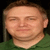## Lotus notes formulaTags:
Lotus Notes
Lotus Notes Formulas
When I use computed text with formula of a multivalue field. it terminates value if semicolon ; is found in the field value. Any suggestion/solution to this problem. I mean to say for ex. abs is a multivalue field and computed text is having formula abc. Then if a value is entered in the field abc as "shjfdsh;sdhfsdjh hjsdf " then in computed text only text "shjfdsh" is showing and rest of the string after ; is terminated. How to solve this used computed field instead of computed text. if it is required to show in computed text then how can this be achieved?

Software/Hardware used:
lotus notes

Thanks. We'll let you know when a new response is added.

Computed text fields don’t understand multivalue fields, you must use the @Implode funtion to make the values a text string.

Example, if text list is the following in the “color” field:
Red
Blue
Green

Then the formula is:

@Implode(color; “; “)

To build a string of:

Red; Blue; Green

## Discuss This Question: 5 Replies

Thanks. We'll let you know when a new response is added.
• Suppose the string is given as REd;blue;;green how do you use the formula to return the exact string.in computed text
report
• If that's the input, what exactly do you want the output to be?  Use your own example.
report
• Input in the field : red;green;blue;;
out put in the computed text:  red;green;blue;;
exactly same.
Input could be any thing including ;,: newline etc
out put in the computed field should be same.
@Implode does not work.
report
• If the input could be anything, then let's take a look at the field that holds the input. If it's multivalue, then look at the properties, 3rd tab - what do you have selected for "Separate values when user enters" and "Display separate values with"?
report
•@Shivasnjay - @Implode will work, but you are looking for it to weed out empty values, to do this you have to add 2 extra steps. And there are 2 ways to do it. If it is just empty values, then use:

imp := @Implode(color; ";");
exp := @Explode(imp; ";"; 0);
@Implode(exp; "; ");

However, if the values may be empty or other characters like @Newline, then you have to use a slightly different approach. My recommendation would be to use the stuff above to get rid of empty strings, and then add additional code like the following:

imp := @Implode(color; ";");
rs := @ReplaceSubstring(imp; @NewLine; "");
exp := @Explode(rs; ";"; 0);
@Implode(exp; "; ");

In the rs variable you could eliminate any other values by setting them to "", then the explode will remove those items and implode will only build a string from what is left.
report